## ASCII Character set in Google Docs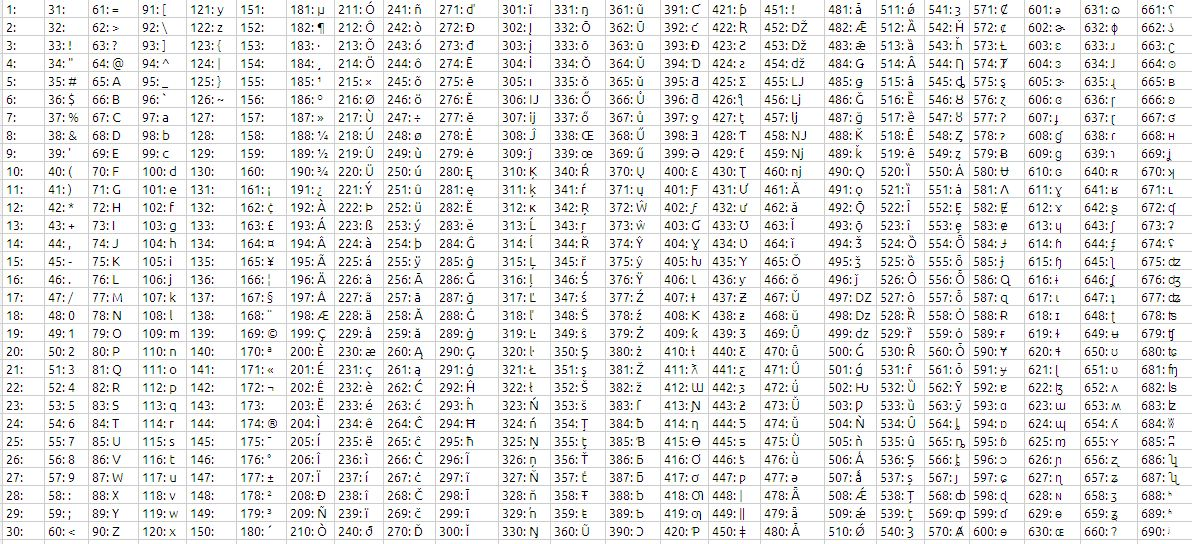The formula used to generate this character set is as follows:

=ARRAYFORMULA(ROW(1:30)+(COLUMN(A:AC)*30-30)&”: “&CHAR(ROW(1:30)+(COLUMN(A:AC)*30-30)))

For each Column you will have 30 characters

the format in each cell is number followed by ASCII character

## IF FUNCTION

The IF function returns one of two values depending on whether a specified expression evaluates to a Boolean value of TRUE or FALSE.

IF(test, then_value, otherwise_value)

test:  A logical expression. test can contain anything as long as the expression can be evaluated as a Boolean. If the expression evaluates to a number, 0 is considered to be FALSE, and any other number is considered to be TRUE.

then_value:  The value returned if the expression is TRUE. if-true can contain any value type. If omitted (comma but no value), IF will return 0.

otherwise_value:  An optional argument specifying the value returned if the expression is FALSE. if-false can contain any value type. If omitted (comma but no value), IF will return 0. If entirely omitted (no comma after if-false) and if-expression evaluates to FALSE, IF will return FALSE.

Usage Notes

(1) If the Boolean value of test is TRUE, the function returns the then_value; otherwise it returns the otherwise_value. Both then_value and otherwise_value can contain additional IF functions (nested IF functions).

(2) the then_value & otherwise_value is optional argument. if left it still the IF function works. if the test results in 1/0 or TRUE/FALSE the result displayed by the function is TRUE/FALSE depending on the test value evaluated.

What is Boolean:

A Boolean value is one that can be expressed in only one of two values, as either TRUE or as FALSE. A FALSE value also has the value of 0. In this case, any other numeric value is considered TRUE. Boolean values are mostly used in comparisons.

Lets understand the IF function with an examples

Exp:1

If i order up to 500 units of the a product, I pay 10.00 per unit. if order from 501 through 1200 units, i pay 9.00 per unit. if i order from 1201 through 2000 units, i pay 8 per unit. if i order more than 2000 units, i pay 7 per unit. How can i write formula that expresses the purchase cost as a function of the number of units purchased?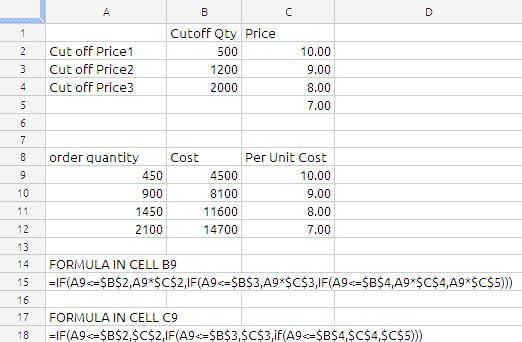we have used the nested IF conditions as shown in above image.

Exp 2:

If the value in Cell A1 is Red than value in the cell B1 will be 25 , if it is Blue then B1 has to be 50 and if A1 is Green then value in B1 has to be 75.

Solution: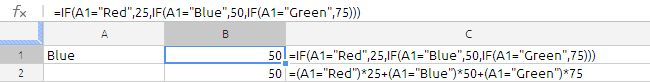in this case we have used two methods using IF function and using boolean algebra, Some time this type of situation using of boolean algebra will much more understandable and it is not constrained by IF function limitations.

This simple comparison will result in a value of TRUE if A2 has “Red” in it (just to be clear: the string of letters, not the background color). If there is anything else or even nothing in A2, then the result will be FALSE. True and False are actual values in the spreadsheet. If you were to enter this formula in some cell, you would see the word TRUE or the word FALSE in that cell, depending on what was in A2.

the spreadsheet is evaluated as shown below:

=(False)*25 + (True)*50 + (False)*75

= (0)*25+(1)*50+(0)*75

=0+50+0

=50

the other method of evaluating this is by use of CHOOSE function, the formula will be

=choose(MATCH(A1,F1:F3,0),25,50,75)

where F1 to F3 will hold Red,Blue, Green

we can also use the vlookup

The IF function has endless uses so we cannot document everything in one article. so i am concluding this article.

## SERIES GENERATION IN GOOGLE DOCS

Question in the Forum:

i need to use data in a column and add two different text strings to each item. this then must be put into a row.
so if my data is (a,b,c,d,) i want the output in my row to be:    | a1 | a2 | b1 | b2 | c1 | c2 | d1 | d2 |.
i have used =TRANSPOSE(ARRAYFORMULA(A2:A5 & ” 1″)) to get | a1 | b1 | c1 | d1 |   but i can’t figure out how to also add the other string.

Suggested solution:

the solution suggested by me is as follows:

=CHAR(64+CEILING(COLUMNS(\$A1:A1),2)/2)&IF(MOD(COLUMNS(\$A1:A1),2)=0,2,MOD(COLUMNS(\$A1:A1),2))

now lets break down the formula

our first formula is

=COLUMNS(\$A1:A1)

if we drag this formula across the columns we will be as shown below image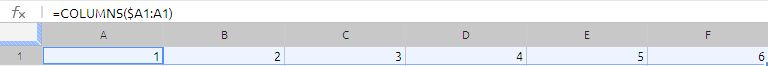since \$A1 is relative reference with column locked this will act as expanding range and will generate the series of numbers from 1,2,3,4,5,6…. etc.

but our objective is to generate the series as 1,1,2,2,3,3… etc.  to convert the 1,2,3,4,5,6…etc into required series we have wrapped this in to CEILING function. now the formula is

=CEILING(COLUMNS(\$A1:A1),2)/2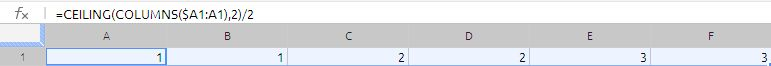now we got the required series, to convert the required series into a alphabet we are using the CHAR function

The formula will be =CHAR(64+CEILING(COLUMNS(\$A1:A1),2)/2)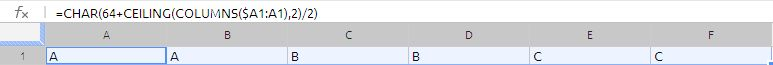Character 65 is A as per Ascii character set, we are using this to convert the number 1,1,2,2,3,3… etc into alphabits A,A,B,B,C,C……….etc

to get the number 1,2,1,2,1,2 we are using the if with MOD function,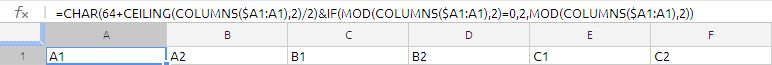With use of MOD function we are converting the odd column numbers to 1 and even column numbers to 2.

## EDATE

The EDATE function returns a date that is “X” number of months before or after a given date.

EDATE(start_date, months)

start-date:  The starting date. start-date is a date value.

months: The number of months before or after the starting date. months is a number value. A negative months is used to specify a number of months before the starting date and a positive months is used to specify a number of months after the starting date.

Example 1:

Suppose we have to issue bank guarantee for a period of 24 month from current date of 13-APR-2012

to calculate the validity period we use the EDATE function as shown in the image below: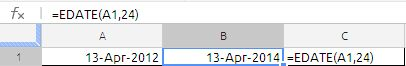Example 2:

the bill due date in 2 months from the current date which is 10-APR-2012 . if the due date happens to be saturday and sunday then the due date will be last Friday.

We can solve this using the Weekday function and edate function. the formulas are clearly explained in the below image.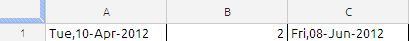the formula in cell C1 is

=EDATE(A1,B1)-IF(WEEKDAY(EDATE(A1,B1),2)>5,WEEKDAY(EDATE(A1,B1),2)-5,0)

The =WEEKDAY() function has been used to identify the actual weekday number of the end date. If the weekday number is 6 or 7, (Sat or Sun), then 5 is subtracted from the =EDATE() to ensure the end of contract falls on a Friday.

## EOMONTH

This function will show the last day of the month which is a specified number of months before or after a given date.

The Syntax of the function is as follows:

EOMONTH(start_date, months)

start-date:  The starting date. start-date is a date/time value.

months: The number of months before or after the starting date. months is a number value. A negative months is used to specify a number of months before the starting date and a positive months is used to specify a number of months after the starting date.

Example 1: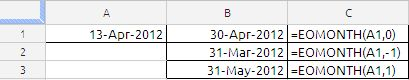Cell A1 contain the start_date, to get to the end of month we have used the 0 in the months argument, Similarly we have used the -1 to get to the end of the previous month.

to get the end of next month from the current date is we are using the 1 in the months argument

## UNIQUE FUNCTION

UNIQUE

Unique function is unique to google docs spreadsheets, the Returns only the unique rows in the source array, duplicates will be ignored

The syntax of the function is as follows:

UNIQUE(SourceArray)

SourceArray:  range of cells, number, date or string, data has to be of the same type, it can also be array formula results.

Note:

• The output of the unique function will in the same order as that of SourceArray.

• unique values will give only for vertical array only

• to get unique values from a horizontal array we have to use the transpose function inside the unique function.

Example 1: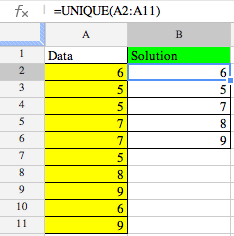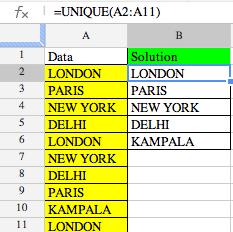the data is cell A2:A11 to extract the unique value from the formula used in cell B2 is

=unique(A2:A1)

Example 2: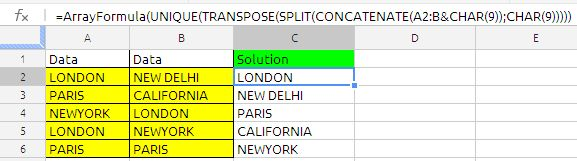we can extract the unique values from column A&B the formula in cell C2 is

=ArrayFormula(UNIQUE(TRANSPOSE(SPLIT(CONCATENATE(A2:B&CHAR(9));CHAR(9)))))

the explanation to the above formula at the following link

https://anandexcels.wordpress.com/2012/02/14/extracting-unique-records-from-two-columns/

## Text Functions

Google Docs spreadsheet has the following text function apart from others

LEFT, RIGHT,MID, TRIM, LEN, FIND, SEARCH, SUBSTITUTE, REPT etc.,

We will see syntax with examples of each function.

LEFT FUNCTION

The LEFT function returns a string consisting of the specified number of characters from the left end of a given string.

LEFT(text, number)

text :  A string. and is  a string value.

number:  An optional argument specifying the desired length of the returned string. string-length is a number value and must be greater than or equal to 1.

Notes

The count includes all spaces, numbers, and special characters.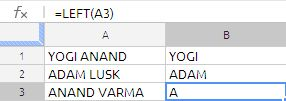the formula in cell B1 is

=LEFT(A1,5)

the formula extracted the first 5 characters of the text string in cell A1 as stated earlier in the notes it has counted the space also.

the formula in cell B3 is

=LEFT(A3)

we have ignored the second argument that means only one character will be extracted

RIGHT FUNCTION

The RIGHT function returns a string consisting of the specified number of characters from the right end of a given string.

RIGHT(text, number)

text:  A string. source-string is a string value.

number:  An optional argument specifying the desired length of the returned string. string-length is a number value and must be greater than or equal to 1.

Notes

If string-length is greater than or equal to the length of source-string, the string

returned is equal to source-string.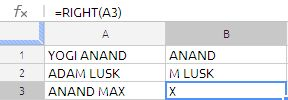the formula in cell B1 is

=RIGHT(A1,6)

the formula extracted the first 6 characters of the text string in cell A1 as stated earlier in the notes it has counted the space also.

the formula in cell B3 is

=RIGHT(A3)

we have ignored the second argument that means only one character will be extracted

MID FUNCTION

The MID function returns a string consisting of the given number of characters from a string starting at the specified position.

MID(text, start, number)

text :  A string. source-string is a string value.

start:  The position within the specified string at which the action should begin. start is a number value that must be greater than or equal to 1 and less than or equal to the number of characters in source-string.

number:  The desired length of the returned string. string-length is a number

value and must be greater than or equal to 1.

Notes

If number is greater than or equal to the length of text, the string returned is equal to text, beginning at start.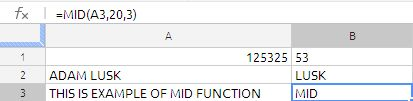The formula in cell B1 is

=MID(A1,3,2)

even though the cell A1 is numeric value still the MID function extract the data but they are in string form that is why the value in the cell B1 is left justified. to convert that string to number we can modify the formula as follows:

=MID(A1,3,2)+0

Similarly the formula in cell B2 is

=MID(A2,6,4)

and formula in cell B3 is

=MID(A3,20,3)

MID function is extensively used along with search, find to extract text.

TRIM FUNCTION

The TRIM function returns a string based on a given string, after removing extra spaces.

TRIM(text)

text:  A string. source-string is a string value.

Notes

TRIM removes all spaces before the first character, all spaces after the last character, and all duplicate spaces between characters, leaving only single spaces between words.

This function is very useful whenever we are importing CSV files into google docs and cleaning the leading and trailing spaces in the string

LEN FUNCTION

The LEN function returns the number of characters in a string. The LEN function very frequently used in google docs spreadsheets when every there is a array formula. it is application is very unique to google spreadsheets.

LEN(text)

text:  A string. source-string is a string value.

Notes

The count includes all spaces, numbers, and special characters.

have look at the following formula structure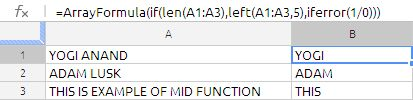in the above formula LEN function is used to restrict the population of the LEFT function to 3 rows.

FIND FUNCTION

The FIND function returns the starting position of one string within another.

FIND(find_text, text, position)

find_text:  The string to find. search-string is a string value.

text:  A string. source-string is a string value.

position:  An optional argument that specifies the position within the specified string at which the action should begin. position is a number value that must be greater than or equal to 1 and less than or equal to the number of characters in find-text.

Notes

The search is case sensitive and spaces are counted. Wildcards are not allowed. To

use wildcards or to ignore case in your search, use the SEARCH function.

SEARCH FUNCTION

The SEARCH function returns the starting position of one string within another, ignoring case and allowing wildcards.

SEARCH(find_text, text, position)

find_text:  The string to find. search-string is a string value.

text :  A string. text is a string value in which we want to search

position:  An optional argument that specifies the position within the specified string at which the action should begin. position is a number value that must be greater than or equal to 1 and less than or equal to the number of characters in source-string.

Notes

Wildcards are permitted in search-string. In search-string, use an * (asterisk) to match multiple characters or a ? (question mark) to match any single character in find_text.

Specifying position permits you to begin the search for text within, rather than at the beginning of, source-string. This is particularly useful if find_text may contain multiple instances of text and you wish to determine the starting

position of other than the first instance. If start-pos is omitted, it is assumed to be 1.

To have case considered in your search, use the FIND function.

Difference Between FIND & SEARCH

1. Both functions find the position of a substring in a string – the position of some characters within a different set of characters.

2. FIND is case sensitive and does not allow wildcards such as * (1 or more characters) or ? a single character.

3. SEARCH is NOT case sensitive and it accepts wildcards.

? Is wildcard for a single character. * Is wildcard for one or more characters.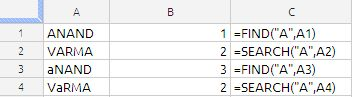in the above image we can clearly see the difference between search and find function in cell B3 & B4,

the Find function failed it identify the “a” in the because it is case sensitive and whereas search could able to identify the “a” position correctly. for all practical purposes we can use the search function instead of find function.

SUBSTITUTE FUNCTION

The SUBSTITUTE function returns a string where the specified characters of a given string have been replaced with a new string.

SUBSTITUTE(text, search_text, new text, occurrence)

text:  A string. source-string is a string value.

search_text:  The string within the given string that is to be replaced. search_text string is a string value.

new text:  The text used as a replacement for the section of the given string that is replaced. new-string is a string value. It does not have to be the same length as the text replaced.

occurrence:  An optional value specifying the occurrence that should be replaced. occurrence is a number value and must be greater than or equal to 1, or omitted. If greater than the number of times existing-string appears within source-string, no replacement will occur. If omitted, all occurrences of existing-string within source string  will be replaced by new-string.

Notes

You can replace individual characters, whole words, or strings of characters within words.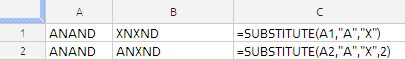in the above example the character “A” is replaced with “X” in cell B1 and in cell B2 we have replaced the second occurrence of “A” has been replaced with “X”.

the substitution function is widely used to extract the certain part of the string along with other function such as Find, Search, MID etc.

Example 1:

Substitute is wide used to count spaces

suppose A1 hold

This  is an example for counting spaces

=LEN(A1)-LEN(SUBSTITUTE(A1,” “,””))

Example 2:

:If we are extracting the text from the 1234567890abcd we have to use the substitute function in other spreadsheet applications the formula will be is as follows:

=substitute(substitute(substitute(substitute(substitute(substitute(substitute(substitute(substitute(SUBSTITUTE(F1,1,””),2,””),3,””),4,””),5,””),6,””),7,””),8,””),9,””),0,””)

fortunately the Google docs spreadsheet supports the regular expression the formula will in cell H1 is

=REGEXREPLACE(F1,”[0-9]”,””)

Example 3: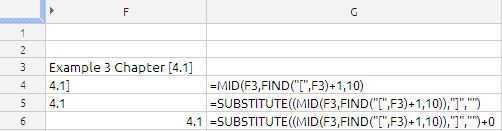Our objective is to extract 4.1 which is in the square brackets.

to extract this we have started with MID function to identify the start point we have used the find function to identify the position and added 1 to avoid the left square bracket

we have removed the right square bracket by using the substitute function by replacing the “]” with a blank

now you can clearly see 4.1 the in Cell F5, but the problem is it is left justified that means it is text.

to convert the text to number we just added 0 (zero) to the formula.

the final formula is

=SUBSTITUTE((MID(F3,FIND(“[“,F3)+1,10)),”]”,””)+0

REPT FUNCTION

The REPT function returns a string that contains a given string repeated a specified number of times.

REPT(text, number)

text:  A string. text is a string value.

number:  The number of times the given string should be repeated. repeat number is a number value that must be greater than or equal to 0.

the REPT function is also used to create a Histogram as shown in the below image.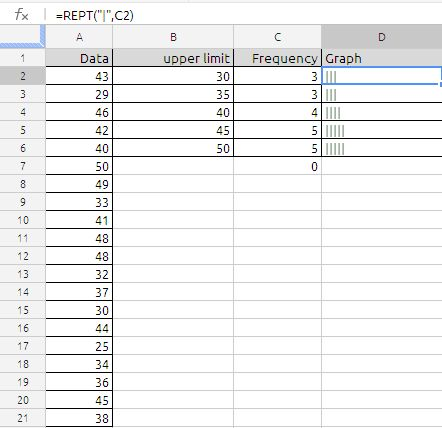Data is in cell A2:A21,

the formula in cell C1 is

=FREQUENCY(A2:A21,B2:B6)

formula in cell D1 is

=REPT(“|”,C2) and drag it down